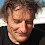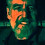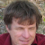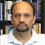## Thursday 2 April 2015

### Joy Christian for dummies (like me)

Over the last decade, Joy Christian has published many articles on his theory that involves a local realistic solution for the EPR type experiment, that is intended to demonstrate entanglement. This solution heavily relies on geometric algebra (GA), a mathematical framework that is not so commonly known. The reason however for using this is that it seems to handle rotations in a more natural way. Furthermore the framework defines a product that does not commute (two objects A and B for which A x B is unequal to B x A), which Joy uses to describe the strong quantum correlations found in EPR type experiments in a local realistic way.

## Geometric Algebra (GA)

Geometric algebra is said to extend the concept of vector algebra. It starts with the definition of orthogonal base vectors, usually called e1, e2 and e3 (here used in 3 dimensions, similar to calling them x, y and z). So any vector can be defined as ae1+be2+ce3, where a, b and c are real numbers. Such a vector can of course as well be written as (a, b, c), which means a times in the e1 direction, b times in the e2 direction and c times in the e3 direction.

### Bivector

Then GA goes on extending the possible objects. Next in line is a bivector, which can somewhat be seen as a combination of two vectors. Is is an oriented part of a plane, and in the same plane as the vectors. The size or weight of the bivector can be calculated as the surface of the parallelogram spanned by the vectors.

The sequence of the vectors indicate the rotational orientation. Therefore the bivector below is different, although constructed from the same vectors.

However a bivector is not a parallelogram. It can as well be drawn as a circle with the same surface. The rotational orientation is now indicated by the lines sticking out from the circle:

To get the bivector spanned by two vectors the wedge product (^) must be used. So when having two vector a and b, a bivector v can be a^b. When the vectors a and b are written in components as

a1e1 + a2e2+a3e3

and

b1e1 + b2e2+b3e3

then the wedge product is

(a1e1 + a2e2+a3e3)^(b1e1 + b2e2+b3e3)

and can be expressed as fractions of the bivectors between the base vectors:

(a1b1-a2b2)(e1^e2) + (a3b1-a1b3)(e3^e1)+(a2b3-a3b2)(e2^e3)

### Trivector

The bivector is a plane, the trivector a volume. It is made of the wedge product between all three of the base vectors: e1^e2^e3.  Now the size of the volume spanned by the three vectors is called the weight. Like the bivecor, the trivector has also rotational orientation (handedness), which is either clockwise or anti-clockwise.

Again it is of no importance how the trivector is drawn. There is no such thing like for example a trivector multiplied in the e1 direction, because one can only multiply its weight.
The same trivector can thus be drawn as sphere, where lines pointing in or outwards indicate the sense of rotational orientation:

The unit trivector, having a weight of 1, is often called 'I' in GA.

### Multivector and operations

In a way, the objects in GA look like calculating with complex numbers or quaternions. A complex number like a+bi, where a and b are real numbers, cannot further be simplified. However one can add two complex numbers by adding their components: (a+bi) + (c+di)= (a+c)+(b+d)i;
The real number, the vector, the bivector and the trivector can all be seen as special cases of a multivector (now a1 to a8 are real numbers):

a1 + a2e1 + a3e2 + a4e3 + a5e1^e2 + a6e2^e3 + a7e3^e1 + a8e1^e2^e3

Another special multivector is the rotor (which is similar to the quaternion):

a1 + a5e1^e2 + a6e2^e3 + a7e3^e1

Adding or subtracting multivectors is by adding or subtracting its components, just like we did with the imaginary numbers. Also its worth remembering that a normal multiplication of one of the base vectors e1, e2 or e3 with itself results in -1, just as  i-squared is in complex numbers.

But in GA there are several types of multiplication between its objects defined.  Here I give an explanation for two ordinary vectors. The product definitions however are usually extended to the complete algebra (1).

Wedge product (a^b):
(a1b1-a2b2)(e1^e2) + (a3b1-a1b3)(e3^e1)+(a2b3-a3b2)(e2^e3)
Outer or cross product (a X b):
(a2b3-a3b2)(e1) + (a1b3-a3b1)(e2)+(a1b2-a2b1)(e3)
Inner product (a.b):
a1b1 + a2b2 +a3b3
Geometric product (ab):
(a1 * b1 + a2 * b2 + a3 * b3)+
(a1 * b2 - a2 * b1)e1^e2 +
(a2 * b3 - a3 * b2)e2^e3 -
(a1 * b3 - a3 * b1)e3^e1

For multivectors some product definitions can be expressed in terms of other product definitions, like:
a b = a . b + a ^ b (for vectors)

Dependent on the grade of the multivector (scalar is grade 0, vector grade 1, bivector grade 2 etc.) the geometric product may or may not be commutative.In the previous post one can see that the non-commutativity between bivectors is responsable for the effect Joys describes in his paper.

I like to conclude by referring again to the program GAViewer (3), which was used to create all the images in this post.

1.Excellent work, Albert-Jan! Now I recommend you work through Christian's one page paper http://arxiv.org/pdf/1103.1879v1.pdf in GAViewer.

I have already implemented it in R+onion. The reason this can be done with quaternions instead of with geometric algebra is because the pure bivectors together with the scalar form a sub-algebra of geometric algebra which is isomorphic to the algebra of the quaternions. And the one-page paper only uses that part of geometric algebra.

2.PS Joy needs more than non-commutativity to obtain the effects which he describes in his paper! He also always needs somewhere to slip in a sign error. This is most clearly seen in the one-page paper, where the error is very close to the surface.

3.This comment has been removed by the author.

4.This comment has been removed by the author.

5.This comment has been removed by the author.

6.Here is the same thing as a GAViewer program: http://www.math.leidenuniv.nl/~gill/test4.g

Notice that I set the random seed at the beginning of the test function:
randSeed(1234);
Comment out this line if you want a different pair of measurement directions with each new test.

I have commented the code, including references to each formula in the one page paper.

7.In order to simulate Christian's earlier and longer paper "Disproof of Bell’s Theorem by Clifford Algebra Valued Local Variables" http://arxiv.org/pdf/quant-ph/0703179v3.pdf in GAViewer, we need to decode Christian's use of the symbol "~/=" in (16).

Let's look at Alice's (alleged) measurement outcome, A_a(mu), where a is an (ordinary) unit vector in R^3, thus an element of S^2. According to the text at formula (14), Bell's hidden variable lambda is replaced by mu = +/-I, where I = e_x ^ e_y ^ e_z. Thus A_a(mu) = +/- I a, B_b(mu) = +/- I b. These are unit length bivectors and the set of unit length bivectors is indeed isomorphic to S^2. Given a and b, each is dichotomous, so we can associate the outcomes +/- I a and +/- I b each with +/- 1. This is exactly what is said in formula (16).

But it still remains to fix the mappings each from (two particular points of) S^2 to {-1, +1}.

Now since the distribution of mu is assumed to be isotropic, all this comes down to the two possible signs having equal probability, and therefore the model has exactly two possible "realisations":

A_a(mu) = - B_b(mu) = +/-1 each with probability 1/2;

and

A_a(mu) = B_b(mu) = +/-1 each with probability 1/2.

According to the first choice the correlation , E(a, b) is identically equal to -1; according to the second choice, E(a, b) is identically equal to +1.

Christian's mistake is to try to calculate the correlation by averaging the product of the two bivectors. Thus he takes the step from (16) to (17) and from there, helped by some more trickery which Albert-Jan has figured out, to (19).

But the two bivectors A_a(mu) and B_b(mu) are just intermediate physical variables which determine "within" each measurement device whether the externally delivered measurement outcome is actually -1 or +1. As Christian indicated through his use of the symbol ~/=, we still have to take the step to a binary outcome coded +/- 1. It is the actual measurement outcomes +/-1 which need to be correlated. The correlations between these outcomes are what is predicted by quantum mechanics, and what is observed in experiments. We do not observe and/or correlate bivectors A_a(mu) and B_b(mu).

Unfortunately, having "decoded" the model of http://arxiv.org/pdf/quant-ph/0703179v3.pdf, simulating it in GAViewer is quite a waste of time.

1.PS When I wrote "A_a(mu) = - B_b(mu) = +/-1 each with probability 1/2" ( and the similar alternative instantiation of the model), both equality symbols "=" should have been equivalence symbols, "~/=".

Equivalence and equality are not the same! In fact, confusing equivalence for equality is exactly what went wrong in Christian's 2007 paper!

8.Hi Albert Jan,

Nice intro to GA. Tomorrow I will post some code for playing around with rotors in GAViewer as soon as I get it cleaned up. It's kind of fun but simple.

I see Gill still hasn't figured GA out yet. Too bad, soooo sad. It really didn't take all that much of an understanding of GA (for me) to see that Joy's model is correct.

1.This was a little more difficult than I was suspecting but here the script for playing around with a basic rotor setup with a slider control. You can keep hitting enter at the prompt for more. Next will be an a and b vector setup with sliders. I think this might be a basis for animating Joy's model somewhat. I hope this script comes out right via html.

// inspired by old DEMOrotor from GABLE
batch DEMOrotor2() {
function unit(a) {
return a / norm(a);
}

//batch init_e3ga();

//init_e3ga();
camori=1;
prompt("DEMOrotor2 >> ");

cmd("label exp_ [5*e1+5*e2] \"\txt{\white{ROTORS} \\ Define a rotation by plane I and angle \phi . \\ Form \eqn{R = e^{-I\phi/2}} \\ All elements X rotate as R \gp A/R.}\" flag_2d flag_px flag_py");
I = white(ori(e1^e2));
ctrl_range(phi=0,-2 pi,2 pi); phi = pi/6;
dynamic{R = white( exp(I phi/2)),}; label(R);
suspend;
cprint("");
R = R, // print it to the console
cprint("");
suspend;
x = e1+e2+e3, label(x);
suspend;
dynamic{Rx = R x/R,}; label(Rx, "R \gp x /R");

suspend;

otherpart = e2+e3;
dynamic{locationA = unit(otherpart) sqrt(-A.A)/ pi;};
dynamic{A = weight(x^otherpart),};
label(locationA,"\white{A}");
suspend;
dynamic{RA = weight(R A/R),};
dynamic{Rlocation = R locationA /R;};
label(Rlocation, "\white{R \gp A /R}");
suspend;
cmd("label exp_ [5*e1+5*e2] \"\txt{\white{ROTORS} \\ Define a rotation by plane I and angle \phi . \\ Form \eqn{R = e^{-I\phi/2}} \\ All elements X rotate as R \gp A/R. \\ \yellow{Do not use the axis, that is soooo 3D.}}\" flag_2d flag_px flag_py");
dynamic{axis = yellow(I phi/I3),}; label(axis,"\yellow{I \phi/I_{3}}");

prompt();
}

2.Ha ha! Show us your GAViewer code of the complete model of http://arxiv.org/pdf/quant-ph/0703179v3.pdf, Fred. Then we might start to imagine that you know something about geometric algebra.

In just a few days, Albert Jan and I (stimulated by Paul Snively) achieved more in that direction than you have done in 8 years.

Trouble is, there are a lot of *words* in Christian's work, and only a few *formulas*. Unfortunately, the words and the formulas do not match. The formulas depend on built-in inconsistencies. As you too will find out, once you start trying to do the job. As we know, Christian doesn't know about computer programming. He also doesn't know about mathematics.

In this connection it is interesting that David Hestenes who not only pioneered and popularized the re-birth of geometric algebra, also came up with a theory of modeling in physics. https://en.wikipedia.org/wiki/David_Hestenes#Modeling_theory_and_instruction The important thing he likes to emphasize is the necessity for the mathematical model to stand on its own two feet, to be itself an object of scientific investigation. This is where Christian has failed - in Hestenes' sense, there is no model! Just some slogans, some imagery, some pseudo-mathematical formalism without meaning. However this kind of sloppy post-modernistic waffling has probably taken over a large part on the one hand of string theory and on the other hand of the foundations of physics. Far from reality (experiments), discipline can break down entirely, physics degenerates into word games.

3.Your code is interesting, Fred. so far I haven't explored the dynamic (interactive) features of GAViewer

4.Hi Albert Jan,

It is actually not my code. It is from an old set of GAViewer tutorial files that I found and modified the DEMOrotor.g file a bit. I will send you the zip file of the old tutorials as soon as I have my computer with GAViewer back online. I'm working on fixing a friend's computer and it is occupying the spot for the GAViewer computer right now. There are some really good GAViewer demos in the set.

5.For those that might be interested, I re-found the link to the old tutorial files.

https://web.archive.org/web/20050821134432/http://www.science.uva.nl/ga/files/20050331_tutorial_files.zip

Enjoy!

6.And here is a link to the old site for GAViewer.

https://web.archive.org/web/20051203083625/http://www.science.uva.nl/ga/index.html

9.Albert Jan will be pleased to hear that Christian is now referring to his GAViewer program in support of his model. See the appendix to "reply to Gill" by J.J. Christian, http://arxiv.org/pdf/1501.03393v3.pdf

Christian refers to Albert Jan's program as an "event-by-event simulation". However, I did not notice any simulation of the binary outcomes A(a, mu) and B(b, mu) ~/= +/- 1 in Albert Jan's program, nor computation of the correlation in the usual way as an average of products of binary (+/- 1) valued outcomes.

We see how important it is that Albert Jan completes his implementation in GAViewer of Christian's 2007 paper http://arxiv.org/pdf/quant-ph/0703179v3.pdf So far, in last month's two posts, Albert Jan has shown that Christian's equation (17) needs to be rewritten in order to get (19) to come out as desired, ie in order to get from LHS of (19) to RHS of (19). But Albert Jan has not yet tackled the definition of the measurement functions A and B in formula (16), and has not yet verified that the correlation of A and B defined in (16) does then coincide with the expression LHS of (19).

There's a lot of work to do! Who will be first: Fred or Albert Jan?

1.A river in Egypt. Apparently Heine got the clue. Spam, spam, spam away.

10.I wrote a "quick-start" introduction to geometric algebra with analysis of two variants of Christian's model: http://www.math.leidenuniv.nl/~gill/GA.pdf . The two versions studied are the Christian's original 2007 four page paper, and the 2011 one page paper. It is interesting to notice how the later paper fills a major gap left in the first and proceeds to fill the gap with an audacious novel definition of correlation for pure bivector valued data.

1.ROTFLMAO! It is pretty pathetic when a dude doesn't even know he has lost the debate. As I said before; you will never understand it no matter how hard you try.

"A man's got to know his limitations". --Dirty Harry

11.By the way, if you really want to know how to rewrite quantum mechanics in terms of geometry, including the EPR-B correlations, take a look at:

http://arxiv.org/abs/quant-ph/0509178

Spacetime algebra and electron physics

C.J.L. Doran, A.N. Lasenby, S.F. Gull, S. Somaroo, A.D. Challinor

It's all there. All, except a local realist model of course ... which we know cannot exist, in view of Bell's theorem.

1.Gill is still living in the dark ages:

http://libertesphilosophica.info/blog/disproof-of-bells-theorem-book/

Perhaps Gill is condemned to live in the dark ages forever.

2.After all this time you would think the poor dude would have a clue. Should I give him another clue?

3.And it is something that won't be found in,

http://arxiv.org/abs/quant-ph/0509178

I know because I have looked high and wide.

12.This comment has been removed by the author.

13.I posted my paper on viXra.

http://vixra.org/abs/1504.0102

1.This comment has been removed by the author.

2.Yeah, Gill is in total denial since Albert Jan proved that your model does in fact produce the result of QM, -a.b. But he will never admit that he has lost the debate because then he would have to pay you the bet he lost. LOL!

3.The original title of the paper was somewhat over the top, and taken as a personal attack. I thought it was quite funny actually, but I guess the moderator was correct, that it was in bad taste.

I would like to know if there are any errors in the content. The paper has been read by quite a few experts, and I got many warm congratulations for its clarity and informativeness.

Still waiting to see whether Fred or Albert Jan will be the first to implement *all* of Christian's model, instead of just half, in GAViewer! I think we will have to wait a long time.

4.By the way, it is nice to see that Fred Diether has now realised that Christian's model is not a local hidden variables model. He writes in the discussion section on my viXra paper "Experiments do +/- 1 valued outcomes. The result of QM's prediction for the correlation of -a.b is not done with +/- 1's. Neither is Dr. Christian's."

Indeed. I wonder if Dr Christian agrees? If so we really are finished: Bell's theorem has not been disproved because Christian's model is *not a local hidden variables model*. He does not model individual measurements. He only models probabilites and expectation values and correlations, just like quantum physics does! Exactly what Einstein objected to!

5.This comment has been removed by the author.

6.And Gill still doesn't know the difference between theory and experiment. How lame is that?

7.It is completely laughable that Gill doesn't know what "local" means after all this time. But I will try to explain one more time in relation to Joy's model for any lurkers out there. The left or right handed orientation of the system of particle pairs happens at creation. Completely local. The a and b vectors are completely random and not in any way connected. Completely local. Yet, the model as now shown to be true via the computer program GAViewer obtains the result of -a.b. It is a shame that Gill's right handed brain can't figure out what a left handed orientation looks like from a right hand only perspective.

8.Fred, why do you think that the a and b vectors are completely random? Experimenters *choose* measurement settings. Alice chooses setting "a" and Bob chooses setting "b". They may make their choices just how they like.

9.Gill is still having trouble telling the difference between theory and experiment. Get real! Get local! LOL!

14.This is worth repeating:

"Experiments do +/- 1 valued outcomes. The result of QM's prediction for the correlation of -a.b is not done with +/- 1's. Neither is Dr. Christian's." [Fred Diether, in the discussion of http://vixra.org/abs/1504.0102]

Fred Diether has seen the light! Christian's "model" is not an event-based model for *individual* binary measurement outcomes. Just like quantum mechanics, it merely predicts correlations.

Since it is has exactly the same "failing" as quantum mechanics itself, one can wonder what the point of the model is, in the light of the fact that David Hestenes, Chris Doran, and others, already rewrote a large part of quantum mechanics in the language of geometric algebra.

What does Dr. Christian think of Diether's current thinking?

1.And Gill does more total misrepresentation as expected. We really don't care if you continue to make a fool of yourself.

2.This comment has been removed by the author.

3.Incredibly high level of discourse here ...

4.This comment has been removed by the author.

5.The only important point in this "discourse" is that the viXra paper Gill has posted above was rejected by the arXiv moderators as a nasty personal attack on me, and for being devoid of any scientific value. In fact it is of *negative* scientific value because it is riddled with schoolboy mathematical and conceptual mistakes. It is extraordinary that after eight years of efforts Gill has yet to learn the first thing about geometric algebra, let alone about my work based on it. It is heartening to know that the arXiv moderators spotted the misleading character of Gill's paper and rejected it at once.

To see Gill's schoolboy howlers (such as his invention of new math where -1 = +1 and LHS not= RHS), the reader may wish to read my already existing arXiv replies to him:

http://arxiv.org/abs/1203.2529

and

http://arxiv.org/abs/1501.03393

For further extensive validation of my work with explicit numerical simulaitons and much more, the reader may also wish to visit my blog: http://libertesphilosophica.info/blog/ .

6.By the way, I have submitted a very long list of derogatory comments by Gill (from literally thousands of his posts on the Internet) to certain authorities, in order to show them just what "level of discourse” Gill routinely engages in.

7."Incredibly high level of discourse here ..."

Incredibly high level of constant misrepresentations here and everywhere else by Gill. He has to know the "game" is over but doesn't want to pay up.